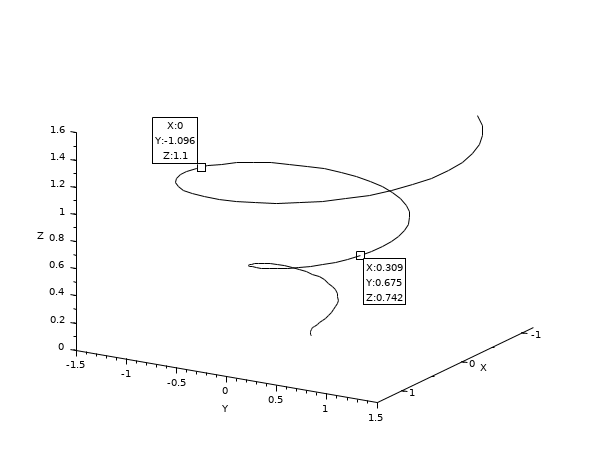Scilab Home page | Wiki | Bug tracker | Forge | Mailing list archives | ATOMS | File exchange
Change language to: English - Français - Português - 日本語 -
Справка Scilab >> Графики > Datatips > datatips

# datatips

Tool for placing and editing tips along the plotted curves

### Description

With this tool, it is possible to place, move and modify tips on the curves (polylines). This can be done by program or interactively. The commonly used mode is the interactive one:

The datatips mode can be enabled by datatipManagerMode() or using the `Edit/Start datatip manager` or clicking on the toolbar iconof the desired graphic window.

Once enabled, a simple left click on a curve will add a tip ( a small box with information on the current point) at the interpolated point of the curve.

To move a tip, press left button on the small square mark on the curve and drag it to the desired location.

To remove a datatip, right click on the small square mark on the curve.

### Examples

```// this examples show how to manage tips by program
x1=linspace(0,1,100)';
y1=x1.^3;
clf();
plot(x1,y1,x1,sinc(10*x1));
e=gce();
p1=e.children(1);//sinc(10*x1)
p2=e.children(2); //x1^3
t=datatipCreate(p1,50);
datatipSetOrientation(t,"lower right")
t=datatipCreate(p1,[0.8 0.5]);
t=datatipCreate(p2,[0.1,0.0]);
t=datatipCreate(p2,[0.8 0.4]);
datatipSetOrientation(t,"upper left")

function str=myfmt(h)
pt = h.data;
str = msprintf('sinc\n%s', sci2exp(round(pt*10)/10))
endfunction
datatipSetDisplay(t,"myfmt")``````// With 3D curves
x1=linspace(0,1.5,100)';
y1=x1.^3;
clf();
param3d(x1.*cos(10*x1),x1.*sin(10*x1),x1);
ax=gca();ax.box='off';
ax.rotation_angles=[76 31];
p=gce();
t=datatipCreate(p,50);
datatipSetOrientation(t,"lower right")
datatipCreate(p,[0,-1.10,1.10]);```